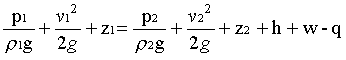### Flow Dirction - Determination

The pressures at two sections of a horizontal pipe are 0.3 kgf/cm2 and 0.6 kgf/cm2 and the diameters are 7.5 cm, and 15 cm respectively. Determine the direction of flow if water flows at a rate of 8.5 kg/sec. State your assumptions.

Data:

P1 = 0.3 kgf/cm2

P2 = 0.6 kgf/cm2

D1 = 7.5 cm

D2 = 15 cm

Mass flow rate = 8.5 kg/sec

Formulae:

• Equation of continuity
• r1A1v1 = r2A2v2

• Bernoulli's equation
• For the flow direction from 1 to 2,• Mass flow rate = volumetric flow rate x density

Calculations:

Volumetric flow rate = 8.5/1000 = 8.5 x 10-3 m3/sec

V1 = 8.5 x 10-3/(pD12/4) = 8.5 x 10-3/(p x 0.0752/4) = 8.5 x 10-3/0.00441= 1.924 m/sec

V2 = 8.5 x 10-3/(pD22/4) = 8.5 x 10-3/(p x 0.152/4) = 8.5 x 10-3/0.01767 = 0.481 m/sec

P1 = 0.3 kgf/cm2 = 0.3 x 9.812 N/cm2 = 2.9436 x 104 N/m2

P2 = 0.6 kgf/cm2 = 0.6 x 9.812 N/cm2 = 5.8872 x 104 N/m2

Assuming the flow direction is from 1 to 2:

2.9436 x 104/1000 + 1.9242/2 = 5.8872 x 104/1000 + 0.4812/2 + h + w -q

29.436 + 1.851 = 58.872 + 0.116 + h + w -q

In the given problem work done by fluid (w) and pump work on fluid (q) are zero.

So to balance the above equation, the quantity h has to have negative values. This is not possible.

The above equation will be a correct one, if the flow is from 2 to 1.

i.e. 58.872 + 0.116 = 29.436 + 1.851 + h

Therefore the flow direction is from the end at which pressure is 0.6 kgf/cm2 and diameter is 15 mm to the end at which pressure is 0.3 kgf/cm2 and diameter is 7.5 mm.

HOME